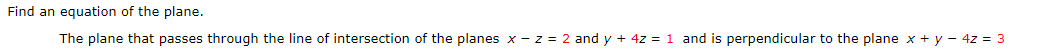Home / Answered Questions / Other / find-an-equation-of-the-plane-the-plane-that-passes-through-the-line-of-intersection-of-the-planes-x-aw390

# (Solved): Find An Equation Of The Plane. The Plane That Passes Through The Line Of Intersection Of The Planes ...Find an equation of the plane. The plane that passes through the line of intersection of the planes x-z = 2 and y + 4z = 1 and is perpendicular to the plane x + y - 4z = 3

We have an Answer from Expert